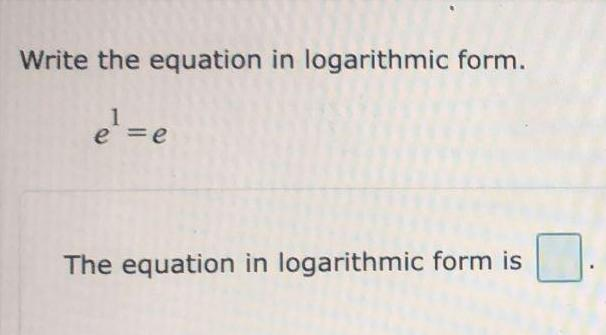Question:

# Write the equation in logarithmic form. e¹=e The equation in

Last updated: 9/1/2022Write the equation in logarithmic form. e¹=e The equation in logarithmic form is